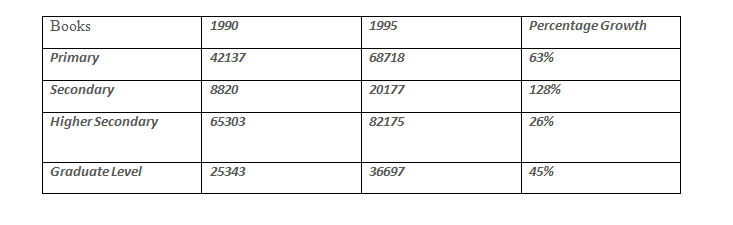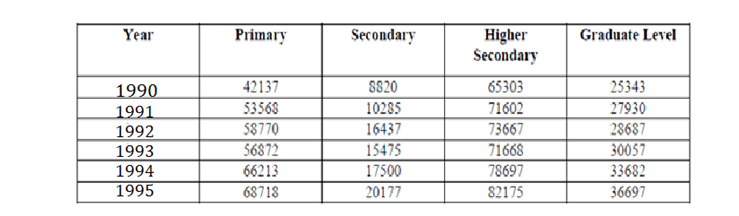DIRECTION : The following table gives the sales details for text books and reference books at Primary/Secondary/Higher Secondary/Graduate Levels.Question 1.  Which of the categories shows the lowest growth rate from 1990 to 1995?
(a) Primary
(b) Secondary
(c) Higher secondary

Question 2.  What is the growth rate of sales of books at primary school level from 1990 to 1995?
(a) 29%
(b) 51%
(c) 63%
(d) 163%

Question 3.  Which of the categories had either a consistent growth or a consistent decline in the period shown?
(a) Primary
(b) Secondary
(c) Higher secondary

Question 4.  Which category had the highest growth rate in the period?
(a) Primary
(b) Secondary
(c) Higher secondary

Solution- From the given table, we may form the following table-From the table it is shown that percentage growth is least for higher secondary books i.e. 26%.

Since we can see that the answer options are not so much closed to each other so we can take the approximate values. so required ratio = (68600 – 42000) 100 / 42000 = 2650 / 42 = 63%